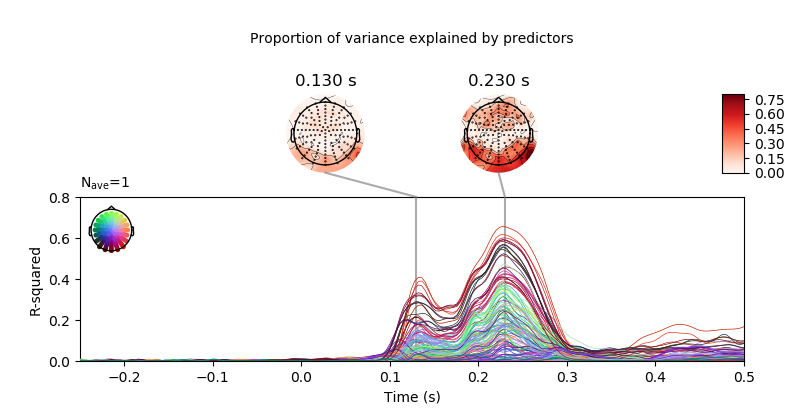# Plot R-squared for a linear model¶

```# Authors: Jose C. Garcia Alanis <alanis.jcg@gmail.com>
#

import numpy as np

from sklearn.linear_model import LinearRegression
from sklearn.metrics import r2_score

from mne.decoding import Vectorizer, get_coef
from mne.datasets import limo
from mne.evoked import EvokedArray
```

Here, we’ll import only one subject. The example shows how to calculate the coefficient of determination (R-squared) for a liner model fitted wit sklearn, showing where (i.e., which electrodes) and when (i.e., at what time of the analysis time window) the model fit best to the data.

```# subject id
subjects = 
# create a dictionary containing participants data
limo_epochs = {str(subj): limo.load_data(subject=subj) for subj in subjects}

# interpolate missing channels
for subject in limo_epochs.values():

# epochs to use for analysis
epochs = limo_epochs['2']

# only keep eeg channels
epochs = epochs.pick_types(eeg=True)

# save epochs information (needed for creating a homologous
# epochs object containing linear regression result)
epochs_info = epochs.info
tmin = epochs.tmin
```

Out:

```1052 matching events found
No baseline correction applied
0 projection items activated
Computing interpolation matrix from 117 sensor positions
Interpolating 11 sensors
```

use epochs metadata to create design matrix for linear regression analyses

```# add intercept
# effect code contrast for categorical variable (i.e., condition a vs. b)
design['face a - face b'] = np.where(design['face'] == 'A', 1, -1)
# create design matrix with named predictors
predictors = ['intercept', 'face a - face b', 'phase-coherence']
design = design[predictors]
```

extract the data that will be used in the analyses

```# get epochs data
data = epochs.get_data()

# number of epochs in data set
n_epochs = data.shape

# number of channels and number of time points in each epoch
# we'll use this information later to bring the results of the
# the linear regression algorithm into an eeg-like format
# (i.e., channels x times points)
n_channels = data.shape
n_times = len(epochs.times)

# vectorize (channel) data for linear regression
Y = Vectorizer().fit_transform(data)
```

fit linear model with sklearn

```# set up model and fit linear model
linear_model = LinearRegression(fit_intercept=False)
linear_model.fit(design, Y)

# extract the coefficients for linear model estimator
betas = get_coef(linear_model, 'coef_')

# calculate coefficient of determination (r-squared)
r_squared = r2_score(Y, linear_model.predict(design), multioutput='raw_values')
# project r-squared back to channels by times space
r_squared = r_squared.reshape((n_channels, n_times))
r_squared = EvokedArray(r_squared, epochs_info, tmin)
```

plot model r-squared

```# only show -250 to 500 ms
ts_args = dict(xlim=(-.25, 0.5),
unit=False,
ylim=dict(eeg=[0, 0.8]))
topomap_args = dict(cmap='Reds', scalings=dict(eeg=1),
vmin=0, vmax=0.8, average=0.05)
# create plot
fig = r_squared.plot_joint(ts_args=ts_args,
topomap_args=topomap_args,
title='Proportion of variance explained by '
'predictors',
times=[.13, .23])
fig.axes.set_ylabel('R-squared')
```Total running time of the script: ( 0 minutes 3.580 seconds)

Gallery generated by Sphinx-Gallery